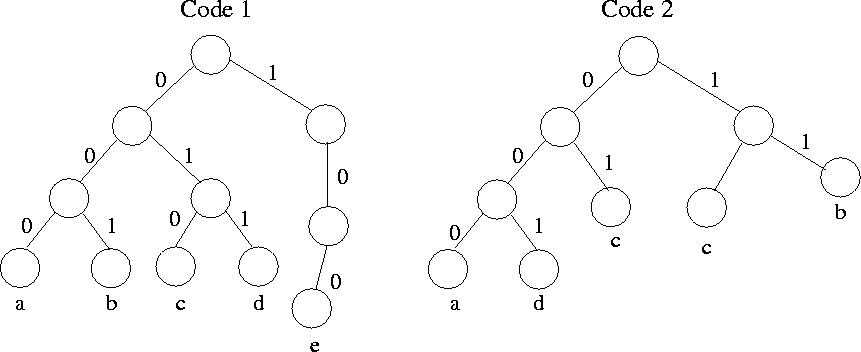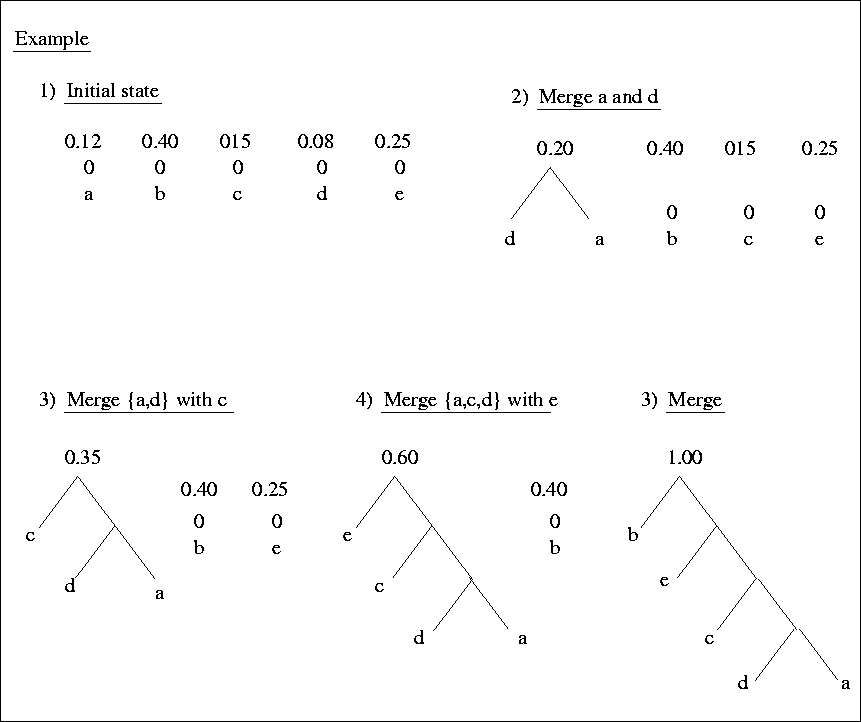Next:4.3.1 ImplementationUp:4. Binary TreesPrevious:4.2 Binary Trees

# 4.3 An Application of Binary Trees: Huffman Code Construction

REF.
David A Huffman. A method for the construction of minimum-redundancy codes. Proceedings of the IRE. Volume 40, Number 9, pp. 1098-1101, 1952.
• Suppose we have messages consisting of sequences of characters. In each message, the characters are independent and appear with a known probability in any given position; the probabilities are the same for all positions.

• e.g. - Suppose we have a message made from the five characters a, b, c, d, e, with probabilities 0.12, 0.40, 0.15, 0.08, 0.25, respectively.

We wish to encode each character into a sequence of 0s and 1s so that no code for a character is the prefix of the code for any other character. This prefix property allows us to decode a string of 0s and 1s by repeatedly deleting prefixes of the string that are codes for characters.

Symbol           Prob          code 1           code 2

a                0.12            000             000

b                0.40            001             11

c                0.15            010             01

d                0.08            011             001

e                 0.25           100             10
In the above, Code 1 has the prefix property. Code 2 also has the prefix property.
• The problem: given a set of characters and their probabilities, find a code with the prefix property such that the average length of a code for a character is a minimum.
• Code 1: (0.12)(3) + (0.4)(3) + (0.15)(3) + (0.08)(3) + (0.25)(3) = 3
Code 2: (0.12)(3) + (0.4)(2) + (0.15)(2) + (0.08)(3) + (0.25)(2) = 2.2
• Huffman's algorithm is one technique for finding optional prefix codes. The algorithm works by selecting two characters a and b having the lowest probabilities and replacing them with a single (imaginary) character, say x, whose probability of occurrence is the sum of probabilities for a and b. We then find an optimal prefix code for this smaller set of characters, using this procedure recursively. The code for the original character set is obtained by using the code for x with a 0appended for ''a'' and a 1 appended for ''b''.
• We can think of prefix codes as paths in binary trees. For example, see Figure 4.8.
•• The prefix property guarantees that no character can have a code that is an interior node, and conversely, labeling the leaves of any binary tree with characters gives us a code with the prefix property for these characters.
• Huffman's algorithm is implemented using a forest (disjoint collection of trees), each of which has its leaves labeled by characters whose codes we desire to select and whose roots are labeled by the sum of the probabilities of all the leaf labels. We call this sum the weight of the tree.
• Initially each character is in a one-node tree by itself and when the algorithm ends, there will be only one tree, with all the characters as its leaves. In this final tree, the path from the root to any leaf represents the code for the label of that leaf.
• The essential step of the algorithm is to select the two trees in the forest that have the smallest weights (break ties arbitrarily). Combine these two trees into one, whose weight is the sum of the weights of the two trees. To combine the trees, we create a new node, which becomes the root and has the roots of the two given trees as left and right children (which is which doesn't matter). This process continues until only one tree remains.
• An example of Huffman algorithm is shown in Figure 4.9 for five alphabets.Next:4.3.1 ImplementationUp:4. Binary TreesPrevious:4.2 Binary Trees
eEL,CSA_Dept,IISc,Bangalore